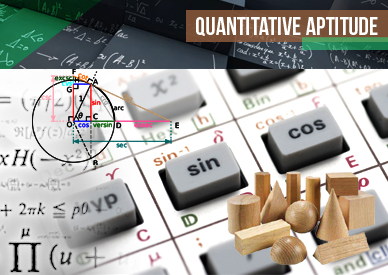## Quadratic equation/Algebra Questions – Set 34

Quadratic equation/Algebra Questions - Set 34 Directions (Q. Nos. 1 to 5) In the following questions, two equations A and…## Algebra For SBI PO : Set – 33

Algebra For SBI PO : Set - 34 D.1-5): In each of these questions, two equations (I) and (II) are…## Algebra For SBI PO : Set – 32

Algebra For SBI PO : Set - 33 D.1-5): In each of these questions, two equations (I) and (II) are…## Algebra For SBI PO : Set – 31

Algebra For SBI PO : Set - 32 D.1-5) : In each of these questions, two equations (I) and (II)…## Algebra For SBI PO : Set – 30

Algebra For SBI PO : Set - 31 D.1-5) : In the following questions two equations numbered I and II…## Algebra For SBI PO : Set – 29

Algebra For SBI PO : Set - 30 D.1-5) : In each of these questions, two equations (I) and (II)…## Algebra For SBI PO : Set – 28

Algebra For SBI PO : Set - 29 D.1-5) : In the following questions, two equations numbered I and H…## Algebra For SBI PO : Set – 27

Algebra For SBI PO : Set - 28 D.1-5) : In each of these questions, two equations (I) and (II)…## Algebra For SBI PO : Set – 26

Algebra For SBI PO : Set - 27 D.1-5) : In each of these questions, two equations (I) and (II)…## Algebra For SBI PO : Set – 25

Algebra For SBI PO : Set - 26 D.1-5): In each of these questions, two equations (I) and (II) are…## Algebra For SBI PO : Set – 24

Algebra For SBI PO : Set - 25 D.1-5): In each of these questions, two equations (I) and (II) are…## Algebra For SBI PO : Set – 23

Algebra For SBI PO : Set - 24 D.1-5): In each of these questions, two equations (I) and (II) are…## Algebra For SBI PO : Set – 22

Algebra For SBI PO : Set - 23 D.1-5): In each of these questions, two equations (I) and (II) are…## Algebra For SBI PO : Set – 22

Algebra For SBI PO : Set - 22 D.1-5): In each of these questions, two equations (I) and (II) are…## Algebra For SBI PO : Set – 21

Algebra For SBI PO : Set - 21 D.1-5): In each of these questions, two equations (I) and (II) are…## Algebra For SBI PO : Set – 20

Algebra For SBI PO : Set - 20 D.1-5): In each of these questions, two equations (I) and (II) are…## Algebra For SBI PO : Set – 19

Algebra For SBI PO : Set - 19 D.1-5) Two equations (I) and (II) are given in each question. On…## Algebra For SBI PO : Set – 18

Algebra For SBI PO : Set - 18 D.1-5) In the following questions two equations numbered I and II are…## Algebra For SBI PO : Set – 17

Directions (Q. 1-5): Two equations (I) and (II) are given in each question. On the basis of these equations you have…## Algebra For SBI PO : Set – 16

Algebra For SBI PO : Set - 16 D.1-5) In the following questions two equations numbered I and II are…## Algebra For SBI PO : Set – 15

Algebra For SBI PO : Set - 15 D.1-5) In each of these questions, two equations (I) and (II) are…## Algebra For SBI PO : Set – 14

Algebra For SBI PO : Set - 14 (1-5) In each of these questions, two equations are given. You have…## Algebra For SBI PO : Set – 13

Algebra For SBI PO : Set - 13 D.1-5) In the following questions, two equations numbered I and II are…## Algebra For SBI PO : Set – 12

Algebra For SBI PO : Set - 12 D.1-6) In the following questions, two equations numbered I and II are…## Algebra For SBI PO : Set – 11

Algebra For SBI PO : Set - 11 D.1-5) In each of these questions, two equation (I) and (II) are…